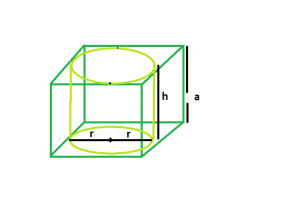Related Articles

# Largest right circular cylinder within a cube

• Last Updated : 17 Mar, 2021

Given a cube of side length a. The task is to find the volume of biggest right circular cylinder that can be inscribed within it.
Examples:

```Input :  a = 4
Output : 50.24

Input : a = 5
Output : 98.125```Approach
Let:

• The height of the cylinder is h.
• Radius of the cylinder be r.

From the diagram it is clear that:

• The height of the cylinder = side of cube
• Radius of the cylinder = side of the cube/2

So,

```h = a
r = a/2```

Below is the implementation of the above approach:

## C++

 `// C++ Program to find the biggest right``// circular cylinder that can be fit within a cube``#include ``using` `namespace` `std;` `// Function to find the biggest right circular cylinder``float` `findVolume(``float` `a)``{``    ``// side cannot be negative``    ``if` `(a < 0)``        ``return` `-1;` `    ``// radius of right circular cylinder``    ``float` `r = a / 2;` `    ``// height of right circular cylinder``    ``float` `h = a;` `    ``// volume of right circular cylinder``    ``float` `V = 3.14 * ``pow``(r, 2) * h;` `    ``return` `V;``}` `// Driver code``int` `main()``{``    ``float` `a = 5;` `    ``cout << findVolume(a) << endl;` `    ``return` `0;``}`

## Java

 `// Java Program to find the biggest right``// circular cylinder that can be fit within a cube` `import` `java.io.*;` `class` `GFG {``  `  `// Function to find the biggest right circular cylinder`` ``static` `float` `findVolume(``float` `a)``{``    ``// side cannot be negative``    ``if` `(a < ``0``)``        ``return` `-``1``;` `    ``// radius of right circular cylinder``    ``float` `r = a / ``2``;` `    ``// height of right circular cylinder``    ``float` `h = a;` `    ``// volume of right circular cylinder``    ``float` `V = (``float``)(``3.14` `* Math.pow(r, ``2``) * h);` `    ``return` `V;``}` `// Driver code`  `    ``public` `static` `void` `main (String[] args) {``            ``float` `a = ``5``;` `    ``System.out.print(findVolume(a));``    ``}``}``// This code is contributed by anuj_67..`

## Python3

 `# Python3 Program to find the biggest``# right circular cylinder that can be``# fit within a cube` `# Function to find the biggest right``# circular cylinder``def` `findVolume(a) :` `    ``# side cannot be negative``    ``if` `(a < ``0``) :``        ``return` `-``1` `    ``# radius of right circular cylinder``    ``r ``=` `a ``/` `2` `    ``# height of right circular cylinder``    ``h ``=` `a` `    ``# volume of right circular cylinder``    ``V ``=` `3.14` `*` `pow``(r, ``2``) ``*` `h` `    ``return` `V` `# Driver code``if` `__name__ ``=``=` `"__main__"` `:` `    ``a ``=` `5` `    ``print``(findVolume(a))` `# This code is contributed by Ryuga`

## C#

 `// C# Program to find the biggest right``// circular cylinder that can be fit within a cube` `using` `System;``class` `GFG {`  `// Function to find the biggest right circular cylinder``static` `float` `findVolume(``float` `a)``{``    ``// side cannot be negative``    ``if` `(a < 0)``        ``return` `-1;` `    ``// radius of right circular cylinder``    ``float` `r = a / 2;` `    ``// height of right circular cylinder``    ``float` `h = a;` `    ``// volume of right circular cylinder``    ``float` `V = (``float``)(3.14 * Math.Pow(r, 2) * h);` `    ``return` `V;``}` `// Driver code`  `    ``public` `static` `void` `Main () {``            ``float` `a = 5;` `   ``Console.WriteLine(findVolume(a));``    ``}``}``// This code is contributed by anuj_67..`

## PHP

 `

## Javascript

 ``
Output:
`98.125`

Attention reader! Don’t stop learning now. Get hold of all the important DSA concepts with the DSA Self Paced Course at a student-friendly price and become industry ready.  To complete your preparation from learning a language to DS Algo and many more,  please refer Complete Interview Preparation Course.

In case you wish to attend live classes with experts, please refer DSA Live Classes for Working Professionals and Competitive Programming Live for Students.

My Personal Notes arrow_drop_up